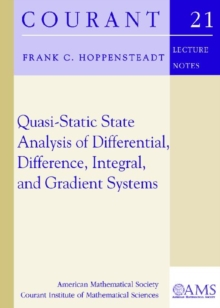Supporting your high street Find out how »
• My Account# Quasi-Static State Analysis of Differential, Difference, Integral and Gradient Systems Paperback / softback

## Part of the Courant Lecture Notes series

#### Description

This book is based on a course on advanced topics in differential equations given in Spring 2010 at the Courant Institute of Mathematical Sciences.

It describes aspects of mathematical modeling, analysis, computer simulation, and visualization in the mathematical sciences and engineering that involve singular perturbations.

There is a large literature devoted to singular perturbation methods for ordinary and partial differential equations, but there are not many studies that deal with difference equations, Volterra integral equations, and purely nonlinear gradient systems where there is no dominant linear part.

Designed for a one-semester course for students in applied mathematics, it is the purpose of this book to present sufficient rigorous methods and examples to position the reader to investigate singular perturbation problems in such equations.

Titles in this series are co-published with the Courant Institute of Mathematical Sciences at New York University.|This book is based on a course on advanced topics in differential equations given in Spring 2010 at the Courant Institute of Mathematical Sciences.

It describes aspects of mathematical modeling, analysis, computer simulation, and visualization in the mathematical sciences and engineering that involve singular perturbations.

There is a large literature devoted to singular perturbation methods for ordinary and partial differential equations, but there are not many studies that deal with difference equations, Volterra integral equations, and purely nonlinear gradient systems where there is no dominant linear part.

Designed for a one-semester course for students in applied mathematics, it is the purpose of this book to present sufficient rigorous methods and examples to position the reader to investigate singular perturbation problems in such equations.

Titles in this series are co-published with the Courant Institute of Mathematical Sciences at New York University.

#### Information

• Format: Paperback / softback
• Pages: 163 pages
• Publisher: American Mathematical Society
• Publication Date:
• Category: Calculus & mathematical analysis
• ISBN: 9780821852699

£34.50

on all orders

###### Pick up orders

from local bookshops

£69.99

£57.89

£119.99

£104.05

£34.50

£119.99

£104.05

£57.50

£57.45

£94.50

£93.55

£29.95

£29.39

£29.50

£29.05